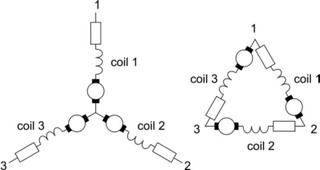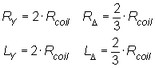﻿ 20-sim webhelp > Toolboxes > Mechatronics Toolbox > Servo Motor Editor > Theory > Basic Principles > Star and Delta Networks

# Star and Delta Networks

Navigation:  Toolboxes > Mechatronics Toolbox > Servo Motor Editor > Theory > Basic Principles >

# Star and Delta NetworksBrushless DC motors and AC synchronous motors use three phase currents to connect to three groups of coils. The connection can be made use a star network or a delta network. Compared to star networks, in a delta network less voltage and more current is needed to produce the same amount of torque. For amplifiers the maximum current is directly related to the costs. That is why delta networks are only used in special cases where higher speeds than normal are required.In 20-sim the electrical resistance and inductance are measured between the terminals (e.g. between 1 and 2 or 2 and 3 or 3 an 1). This is known as the terminal resistance and inductance or as the phase to phase resistance and inductance. There are two reasons for using terminal values. Most motor suppliers give the terminal values in their data sheet and by using terminal values the same dynamic model can be used for star networks and delta networks.

If the motor supplier gives coil values instead of terminal values, you have to calculate them by hand. The relation between the terminal values and the coil values is: